# Riemannian Geometry

Also found in: Dictionary, Thesaurus, Wikipedia.

## Riemannian geometry

[rē′män·ē·ən jē′äm·ə·trē]
(mathematics)
McGraw-Hill Dictionary of Scientific & Technical Terms, 6E, Copyright © 2003 by The McGraw-Hill Companies, Inc.
The following article is from The Great Soviet Encyclopedia (1979). It might be outdated or ideologically biased.

## Riemannian Geometry

a multidimensional generalization of the geometry on a surface. It is the theory of Riemannian spaces, that is, spaces in which Euclidean geometry holds in the small. Riemannian geometry is named after B. Riemann, who set forth its fundamentals in 1854.

Introduction. When a smooth surface in Euclidean space is considered from the standpoint of measurements made on it, it turns out to be a two-dimensional space whose intrinsic geometry is not precisely Euclidean. The geometry of the surface is, however, Euclidean in the small—that is, in the neighborhood of any point the geometry coincides, up to infinitesimals of higher order, with the geometry of the tangent plane. In addition, such a surface is generally nonhomogeneous in its geometric properties. The intrinsic geometry of the surface is therefore a Riemannian geometry of two dimensions, and the surface is a two-dimensional Riemannian space.

Thus, for segments of the earth’s surface that are small compared with the dimensions of the earth, measurements can be successfully based on ordinary plane geometry. The results of measurements on large segments, however, exhibit a considerable deviation from the laws of plane geometry. If we transfer these notions to multidimensional spaces, we obtain general Riemannian geometry. Three ideas underlie Riemannian geometry. The first idea was originally developed by N. I. Lobachevskii and consists in admitting the very possibility of the existence of a geometry differing from Euclidean geometry. The second idea is K. F. Gauss’ concept of the intrinsic geometry of surfaces along with its analytic apparatus as embodied in the quadratic form that determines the line element of the surface. The third idea is the concept of n-dimensional space, which was advanced and elaborated in the first half of the 19th century by a number of geometers. Riemann combined and generalized these ideas in the lecture “Über die Hypothesen, welche der Geometrie zu Grunde liegen” (On the Hypotheses That Form the Foundations of Geometry), which was delivered in 1854 and published in 1867. In this lecture he introduced the general concept of a space as a continuous set of any type of like objects, which are the points of the space; he transferred to these spaces the notions of measurement of length in small steps.

After the publication of Riemann’s works, his ideas drew the attention of a number of mathematicians who further developed the analytic apparatus of Riemannian geometry and established new results of geometric significance. An important step was the invention of tensor calculus by the Italian geometers G. Ricci-Curbastro and T. Levi-Civita at the turn of the 20th century. The tensor calculus proved to be the most suitable analytic apparatus for the elaboration of Riemannian geometry. A. Einstein made use of Riemannian geometry in his general theory of relativity. The creation of this theory was a triumph not only of abstract geometry but also of Lobachevskii’s and Riemann’s ideas on the relation between geometry and physics. Einstein’s application of Riemannian geometry was of decisive importance and led to the vigorous development of Riemannian geometry and its various generalizations. At the present time, Riemannian geometry and its generalizations constitute a broad field of geometry that continues to develop successfully, with special attention being devoted to problems of a global character.

Definition of Riemannian space. A rigorous definition of a Riemannian space may be approached as follows. The position of a point in an n-dimensional manifold is determined by n coordinates x1, x2, …, xn. In n-dimensional Euclidean space, the distance between any two points X and Y in suitably chosen coordinates is expressed by the formula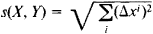where Δxi is the difference between the ith coordinates of X and Y. Correspondingly, in a Riemannian space, coordinates x1, …, xn can be introduced in the neighborhood of every point A so that the distance between points X and Y close to A is expressed by the formula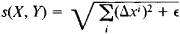where ∊/s(X, Y) → 0 as X and Y approach A. It follows that in arbitrary coordinates the distance between nearby points (xi) and (xi + dxi)— or, equivalently, the differential of the arc length of a curve—is given by the expression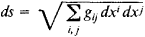where the coefficients gij = gij(x1, …, xn) are functions of the coordinates. This expression is called the line element of Riemannian space. Thus, a Riemannian space R may be analytically defined as an n-dimensional manifold with a differential quadratic form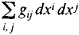defined at every point. This form is also called the metric form, or simply the metric, of R and is by definition positive definite. Since transformations of coordinates are possible, the same Riemannian space has for different coordinates different expressions for the metric form. On the other hand, because of its geometric meaning as the square of the element of arc length, the value of the form must remain invariant under a transformation of coordinates from xi to i; that is,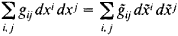WE thus have a specific law of transformation of the coefficients gij. The law implies that the coefficients gij are components of a covariant tensor of order two called the metric tensor of the Riemannian space.

With every point A of a Riemannian space R there is associated a tangent Euclidean space EA into which some neighborhood U of A is mapped in such a way that the relative distortion of distances tends to zero as A is approached. Analytically, this reduces to the introduction, near a point A0 in EA, of coordinates such that the square of the linear element ds20 of EA is expressed at A0 by the same form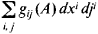that expresses the square of the linear element ds2 of the Riemannian space at A. Thus, neglecting infinitesimals of order higher than one, we may replace the neighborhood of a point in a Riemannian space by the neighborhood of a point in its tangent space.

Basic concepts of Riemannian geometry. (1) The arc length s of the curve xi = xi(t) (i = 1, …, n, t1tt2) is defined as the integral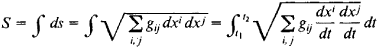along the curve. As Riemann remarked, this corresponds, in a sense, to measuring lengths “in terms of a small unit.” If any two points of R can be connected by a curve, then R is a metric space. The distance ρ (X, Y) between the two points is defined as the greatest lower bound of the lengths of the curves joining the points and is called the intrinsic metric of the Riemannian space R.

(2) The angle between two curves issuing from the same point A is defined as the angle between the tangent vectors to the curves at A.

(3) The volume V of an n-dimensional region G of a Riemannian space is defined by the formula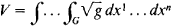where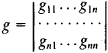Geodesics. The curves that, in sufficiently small regions, are the shortest of all curves having the same ends are called geodesics. They play the role of straight lines in the Riemannian space R. By definition they are the extremals of the functional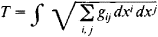(seeCALCULUS OF VARIATIONS) and satisfy the equations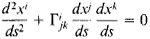where the Γijk are christoffel symbols, which are expressed in terms of the components of the metric tensor gij and their first derivatives. Through every point of the Riemannian space in any direction there passes a geodesic. Any two points A and B of a sufficiently small region can be joined by a unique shortest curve, whose length is equal to the intrinsic distance ρ (A, B) between the points. The uniqueness may be violated, however, if the points are sufficiently distant from each other; for example, the poles of a sphere can be connected by an infinite set of arcs of great circles, which are geodesies.

The estimation of the number v of closed geodesics of a space R is of interest, for example, for the description of periodic motions in the many-body problem of mechanics. The question of the value of v was posed by J. H. Poincaré in 1905 in connection with certain problems of celestial mechanics but remains far from solution, despite the efforts of many mathematicians. The best result so far is v≥ 2 if R is simply connected.

Osculating space. Between a Riemannian space R and the tangent Euclidean space in the neighborhood U of some point A there can be established a correspondence such that both spaces will coincide to within infinitesimals of higher than second order. For this purpose, geodesies are drawn in all directions from A, and with each of them there is associated in the tangent space a ray of corresponding direction. A correspondence between these rays and geodesies is then established such that the lengths of the arcs of the geodesies and of the corresponding rays are equal. In a sufficiently small neighborhood, such a correspondence is one-to-one. If Cartesian coordinates x1, … xn are introduced in the tangent space and their values are assigned to the corresponding points of U, then the following relation holds between the line elements ds of the Riemannian space and ds0 of the Euclidean space: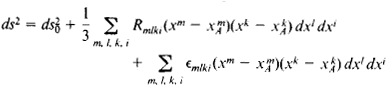where ∊mlki → 0 as xixiA, i = 1, …, n. It follows that the difference ds - ds0 is of the order at least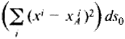A Euclidean space placed in such a correspondence with a Riemannian space is called an osculating space, as distinguished from an ordinary tangent space. In the general case, it is impossible to achieve a higher order of coincidence by special selection of the correspondence between the Riemannian and Euclidean spaces. The coefficients Rmlki therefore characterize the difference between the Riemannian space and the Euclidean space. These coefficients are the components of what is known as the Riemann-Christoffel curvature tensor, which is defined by the formula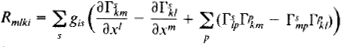only in terms of the gik and their derivatives up to second order.

The identical vanishing of the curvature tensor is necessary and sufficient for the space in the neighborhood of each point to coincide with the Euclidean space. In the large, the Riemannian space may differ from the Euclidean space in structure, just as the lateral surface of a cylinder differs from a plane.

Parallel displacement. For any smooth curve L in a Riemannian space there exists a mapping of its neighborhood UL into the Euclidean space EL such that EL is osculating at all points of L. The image of L in EL is called the developable L’ of this curve in the Euclidean space. We note that for a surface F in Euclidean space the osculating Euclidean space along L can be interpreted as the envelope, developed on a plane, of the family of planes tangent to F along L. One says that a vector (and any tensor) undergoes a parallel displacement along L if there occurs a parallel displacement of the corresponding vector (tensor) in the Euclidean space EL osculating with the Riemannian space along this curve. Analytically, the parallel displacement of a vector αi along a curve xi = xi(t) is defined by the differential equation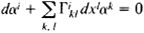If αi= dxi/ds, then we obtain the equation of the geodesies. Thus, geodesies may be defined as curves along which the tangent vector undergoes parallel displacement—that is, the developable of a geodesic is a straight line, a circumstance that deepens the similarity of geodesies to straight lines. In general, the result of the parallel displacement of a vector from a point A to a point B depends on the curve AB along which the displacement occurs. Indeed, the difference between a Riemannian space and a Euclidean space shows up visually in this absence of “absolute parallelism.”

Geodesic curvature. The geodesic curvature, or first curvature, of a curve L at a point M is a measure of the deviation of L from the geodesic L0 that is tangent to L at M. Geodesic curvature is defined in the following manner. Suppose the tangent vector to L at M undergoes parallel displacement to the point M, where it forms an angle Φ with the tangent vector to L at M. Let s be the length of the arc MM of L. When M’ approaches M, we obtain the limit

κ = lim Φ/s

This limit is called the geodesic curvature of L at M. Analytically, the geodesic curvature of a curve xi = xi(s) parameterized by the arc length is defined by the formula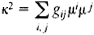where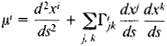Thus, the geodesic curvature of L coincides with the first curvature of its developable Lʹ, and the geodesies have zero geodesic curvature at all their points.

For a curve L in a Riemannian space R, there also are defined second and higher curvatures, and there hold relations analogous to the conventional Frenet formulas (seeDIFFERENTIAL GEOMETRY) for curves in Euclidean space.

Riemannian curvature. Suppose M is a point in a Riemannian space, F is a two-dimensional surface xi = xi(u, v) passing through M, L is a simple closed loop on F passing through M, and σ is the area of the part of the surface bounded by L. Let an arbitrary vector ai tangent to F—that is, expressed linearly in terms of the vectors ∂xi/∂u and ∂xi/∂v—be carried by parallel displacement along L. After the vector has undergone parallel displacement, the vector’s component tangent to F forms with ai an angle ɸ; the positive direction of the measurement of angles here should coincide with the direction in which L is traversed. When L is contracted to M, we obtain the limit

K = lim ɸ/σ

This limit is called the curvature of the Riemannian space, or the Riemannian curvature, at the given point in the direction of the two-dimensional surface. K depends not on the surface but only on its direction at M, that is, on the direction of the two-dimensional plane in the tangent Euclidean space that contains the vectors ∂xi/∂ u and ∂xi/∂v.

The relation between the Riemannian curvature K and the curvature tensor is given by the formula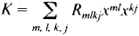where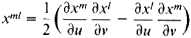Here, the parameters u and v are chosen so that the parallelogram on the vectors ∂xi/∂u and ∂xi/∂v has unit area.

In the two-dimensional case, K coincides with the total curvature—a result expressed in Gauss’ Theorema egregium (Extraordinary Theorem) of 1827. Moreover, for a region G bounded by a simple closed curve having geodesic curvature κ, the Gauss-Bonnet formula holds:

GK dσ + ∫Γ κ ds = 2π

In particular, for a triangle formed by segments of geodesics,

GK dσ = A + B + C – π

where A, B, and C are the magnitudes of the angles of the triangle. In the case of a closed two-dimensional Riemannian space R, that is, a compact space without a boundary, the Euler characteristic χ(R) of R is proportional to the integral of the Riemannian curvature:

2πχ(R) = ∬RK

This formula has been generalized to the case of an even-dimensional closed Riemannian space in which a certain function of the components of the curvature tensor is integrated.

If at every point of a Riemannian space the curvature is independent of the direction of the two-dimensional surface, then the curvature does not vary from point to point—that is, the space has constant curvature. Riemannian spaces having a curvature tensor of special structure are also of interest; they are useful, in particular, in describing mechanical systems with cyclic coordinates. Such spaces are a generalization of spaces of constant curvature and have a large group of motions. Examples of such spaces are symmetric spaces, whose curvature tensor does not vary under parallel displacement and subprojective spaces, which are characterized by a special coordinate system in which geodesics are described by linear equations.

Riemannian curvature plays an important role in the geometric applications of Riemannian geometry, especially since a Riemannian metric can be introduced on any manifold. For example, the topological structure of complete Riemannian spaces—that is, spaces in which any geodesic is infinitely extendible—depends on the properties of the curvature of the space. In particular, any complete simply connected n-dimensional Riemannian space is homeomorphic to an n-dimensional Euclidean space if its curvature at all points and in all directions is nonpositive and to an n-dimensional sphere of unit radius if its curvature K satisfies the inequalities 1 ≥ K1/δ > ¼, where δ is a constant. The diameter d of a complete Riemannian space R is the least upper bound of the distances between the points of R as determined by the intrinsic metric of R. This diameter also depends on the value of the curvature of the space. For example, if KK0 > 0, then d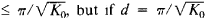, then R is a sphere of radius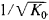Metric connection. A parallel displacement along a curve L having ends A and B determines an isometric, that is, distance-preserving, transformation τl, of the tangent space EA at A into the tangent space EB at the point B. The differential of the transformation τl, at A—that is, the principal linear part of the change in τl when we move from A (xi) to a nearby point Ã(xi + dxi)—determines a geometric entity that is called the Riemannian connection associated with the given parallel displacement. Analytically, this connection is expressed by the system of linear differential forms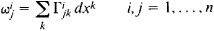In a Riemannian space R we can also define other connections such that the associated parallel displacements preserve the metric tensor. Such connections are called metric connections and are defined by analogous coefficients Γijk but they are not symmetric with respect to the indices and k and are not expressed (like Christoffel symbols) only in terms of the tensor gij and its derivatives. The difference between a metric connection and a Riemannian connection is represented by the torsion tensor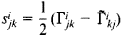whose geometric meaning can be illustrated in the following manner. Let us consider, in a two-dimensional Riemannian space of metric connection, a small triangle formed by segments of geodesics of length a, b, and c and by angles A, B, and C. Then the principal part of the projection of the torsion at A in the direction of AB is equal to the ratio of the quantity c - a cos B - b cos A to the area of the triangle, and the principal part of the projection of the torsion on the perpendicular to AB is equal to the quantity a sin B - b sin A divided by the area of the triangle. Thus, in a Riemannian space of zero torsion the cosine and sine theorems of ordinary trigonometry hold up to quantities that are small compared with the area of the triangle.

Curves whose tangent vector undergoes parallel displacement along them are called geodesies of the corresponding connection. Such curves coincide with Riemannian geodesies if the tensor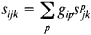is skew-symmetric with respect to all indices.

Subspaces. On the m-dimensional submanifold M of a Riemannian space R defined by the equations xi = xi (ul, …, um), where the rank of the matrix ∥∂xi/∂uα∥ is equal to m, there holds the Riemannian geometry defined by the metric tensor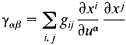M is called a Riemannian subspace of R.

A sufficiently small region of the m-dimensional Riemannian space R can be imbedded in a Euclidean space of sufficiently large dimension N— that is, the region admits a length-preserving mapping onto a submanifold of this space. It is known that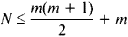The question of the minimum value of N in the general case remains unsolved. If, however, the coefficients of the metric form gij of R are analytic functions—that is, can be represented by convergent power series—then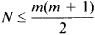Even less is known about imbedding in the large, which is a problem relevant to the physics of gauge fields.

Imbeddings of two-dimensional Riemannian spaces have been investigated in greatest detail. Some examples follow.

(1) A two-dimensional complete Riemannian space of positive curvature K can be imbedded in the form of a closed convex surface, or ovaloid, in a three-dimensional Riemannian space of curvature no less than K. This problem was posed by H. Weyl in 1916 and was solved by the German mathematician H. Levy in 1937 and by A. D. Aleksandrov in 1941 for imbedding in a Euclidean space and by A. V. Pogorelov in 1957 for a Riemannian space. In addition, any two imbeddings having a common point and a common osculating surface at this point coincide—that is, an ovaloid is uniquely defined by its metric. Important results in this connection were obtained by the German mathematician S. E. Cohn-Vossen in 1927 and by Pogorelov in 1948.

(2) The Soviet mathematician N. V. Efimov showed in 1963 that a two-dimensional complete Riemannian space of negative curvature K ≤ K0< 0 does not admit an imbedding in the form of a regular surface. The special case of a Lobachevskian plane (K = – 1) was analyzed by D. Hilbert in 1901.

(3) The Soviet mathematician E. G. Pozniak proved in 1973 that a two-dimensional Riemannian space homeomorphic to a torus admits an imbedding in a four-dimensional Euclidean space.

Applications and generalizations. Since a Riemannian geometry is defined by the assignment of a symmetric covariant tensor of order two, any physical problem that reduces to the study of such a tensor field can be formulated as a problem of Riemannian geometry. In particular, tensor fields of this type include various physical quantities that characterize the elastic, optical, thermodynamic, dielectric, piezomagnetic, and other properties of anisotropic bodies. The symmetry of the coefficients gij here is a reflection of one of the fundamental laws of physics—the reciprocity principle. Riemannian geometry found its first application in Riemann’s solution in 1861 of the problem of heat conduction in an anisotropic body.

The study of configuration space in the mechanics of a system with n degrees of freedom has permitted a number of mechanical problems to be presented in a clear geometric form. For example, the trajectories of the free motion—that is, motion in the absence of generalized forces—of a holonomic mechanical system of kinetic energy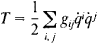where the q̇i generalized velocities, are the geodesics of the corresponding n-dimensional Riemannian space with the metric tensor gij. Some other facts were mentioned above. An analogous interpretation has also been given to motion in a field of forces having a potential (seeLEAST CURVATURE, PRINCIPLE OF).

In applications of Riemannian geometry to mechanics and physics an important role is played by additional structures that are consistent, in some sense, with the metric of a Riemannian space. Examples follow.

(1) To physical concepts of an elastic continuous medium with continuous distribution of the sources of internal stresses there corresponds a Riemannian space with a certain metric connection. The parallel displacement corresponding to the connection determines the natural state of the medium along the curve, and the torsion is identified with the dislocation density.

(2) Quantum mechanics uses Riemannian spaces of nearly complex structure—that is, spaces determined by the field of the covariant and contravariant tensor Jlk of order one such that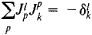where δlk is the Kronecker delta—to describe the observed states of many-particle systems.

(3) The use of the concept of conformai connection—that is, a connection of Riemannian space in which the result of the parallel displacement of the metric tensor gij is proportional to the tensor—has permitted the modeling of some of Bohr’s postulates. In particular, the allowed orbits of electrons in an atom are curves along which the metric tensor is preserved.

The development of Riemannian geometry in connection with the general theory of relativity and continuum mechanics gave rise to various generalizations of its content, the most important of which are the pseudo-Riemannian spaces. An example of such a space is, according to the theory of gravitation, the space-time manifold, which is a four-dimensional space on which there is defined the alternating nondegenerate quadratic form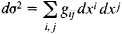It is the coefficients of this “metric,” which permit imaginary distances, that characterize the gravitational field; the coefficients play the role of potential functions. At each point of the space-time manifold, this form can be reduced to the form

2 = dx2 + dy2 + dz2 – dt2

where x, y, and z are the space coordinates, and t is the time coordinate. Physically, such locally Galilean frames of reference are freely falling in the gravitational field. Such a system, however, cannot be introduced on the entire manifold, since the presence of the gravitational field is mathematically expressed in the curvature of the pseudo-Riemannian space.

There are other ways of generalizing Riemannian geometry. For example, Finsler geometry makes use of more general laws for the determination of the distances given in the form of the linear elements ds. Other generalizations are based on more general laws of parallel displacement or on the rejection of the requirements of regularity.

### REFERENCES

Riemann, B. Soch. Moscow-Leningrad, 1948. (Translated from German.)
Rashevskii, P. K. Rimanova geometriia i tenzornyi analiz, 3rd ed. Moscow, 1967.
Eisenhart, L. P. Rimanova geometriia. Moscow, 1948. (Translated from English.)
Schouten, J. A. Tenzornyi analiz dlia fizikov. Moscow, 1965. (Translated from English.)
Gromoll, D., W. Klingenberg, and W. Meyer. Rimanova geometriia v tselom. Moscow, 1971. (Translated from German.)

A. D. ALEKSANDROV and IU. F. BORISOV

The Great Soviet Encyclopedia, 3rd Edition (1970-1979). © 2010 The Gale Group, Inc. All rights reserved.
References in periodicals archive ?
JOSHI, Riemannian geometry for the statistical analysis of diffusion tensor data, Signal Process., 87 (2007), pp.
It seems that Smarandache Geometries are connected with the Theory of Relativity (because they include the Riemannian geometry in a subspace) and with the Parallel Universes (because they combine separate spaces into one space only) too.
Hilbert Geometry is a particularly simple metric space on the interior of a compact convex set H [member of] [R.sup.n] that can be used to explore and visualize properties of non-Euclidean and even non Riemannian geometry. It has been proposed by David Hilbert as an example of non- Euclidean geometry for which the Euclidean straight lines are shortest geodesic curves.
This theory has some advantages over the general relativity; the quantities such as christoffel symbols and others become tensors which otherwise in Riemannian geometry they are not.
These map the same reality in different ways, like Euclidean geometry and Riemannian geometry.
MUSIC OF THE SPHERES In the early 1990s, working in the United States, Perelman had emerged as a major player in Riemannian geometry, which studies subjects such as curvature.
 do Carmo, Riemannian Geometry, Birkhauser, 1992.
In Euclidean geometry, the three angles of a triangle add up to 360 degrees; in Lobachevskian geometry, they add up to less than 360 degrees; and in Riemannian geometry, they add up to more than 360 degrees.
In this work, we basically deal with the first-order ODEs and PDEs in the context of Riemannian geometry and Riemannian structure defined on a submanifold corresponding to given equation enables one to give a geometric description of an interesting class of equations in mathematical physics.

Site: Follow: Share:
Open / Close